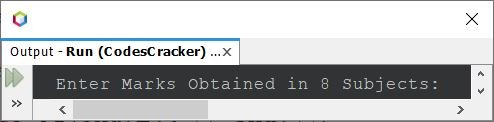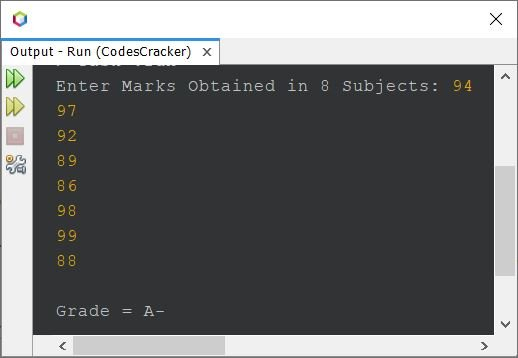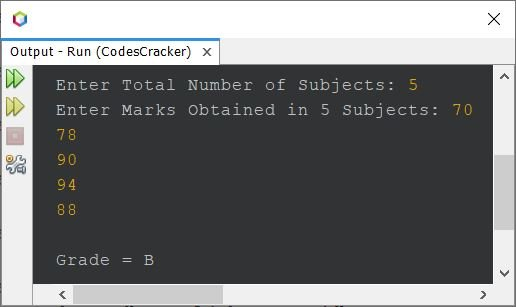# Java Program to Calculate Grades of Student

This post covers a program in Java that find and prints the grade of a student based on the marks entered by user at run-time of the program.

The grades of student must be calculated as per the following table:

>= 94 A
>= 90 and < 94 A-
>= 87 and < 90 B+
>= 83 and < 87 B
>= 80 and < 83 B-
>= 77 and < 80 C+
>= 73 and < 77 C
>= 70 and < 73 C-
>= 67 and < 70 D+
>= 63 and < 67 D
>= 60 and < 63 D-
< 60 F

Note - In above table, the range >= 90 and < 94 means, if the average mark is greater than or equal to 90 and less than 94, then the grade should be A-. Under this range, some marks may lie are 90, 90.23, 92, 93.89 etc. That is, in the range of 90-94 here, 90 is included, whereas 94 is excluded.

## Find Grades of Student in Java - Basic Version

The question is, write a program in Java to find grade of student using if-else. The program given below is its answer:

```import java.util.Scanner;

public class CodesCracker
{
public static void main(String[] args)
{
float[] marks = new float;
float sum=0, avg;
int i;
Scanner scan = new Scanner(System.in);

System.out.print("Enter Marks Obtained in 8 Subjects: ");
for(i=0; i<8; i++)
marks[i] = scan.nextFloat();

for(i=0; i<8; i++)
sum = sum + marks[i];
avg = sum/8;

if(avg>=94)
System.out.println("A");
else if(avg>=90 && avg<94)
System.out.println("A-");
else if(avg>=87 && avg<90)
System.out.println("B+");
else if(avg>=83 && avg<87)
System.out.println("B");
else if(avg>=80 && avg<83)
System.out.println("B-");
else if(avg>=77 && avg<80)
System.out.println("C+");
else if(avg>=73 && avg<77)
System.out.println("C");
else if(avg>=70 && avg<73)
System.out.println("C-");
else if(avg>=67 && avg<70)
System.out.println("D+");
else if(avg>=63 && avg<67)
System.out.println("D");
else if(avg>=60 && avg<63)
System.out.println("D-");
else
System.out.println("F");
}
}```

The snapshot given below shows the initial output produced by above Java program:Now supply the marks obtained in 8 subjects. Here is its sample run, after providing marks say 94, 97, 92, 89, 86, 98, 99, 88 as eight subject marks:The above program can also be created in this way:

```import java.util.Scanner;

public class CodesCracker
{
public static void main(String[] args)
{
float[] marks = new float;
float sum=0;
Scanner scan = new Scanner(System.in);

System.out.print("Enter Marks Obtained in 8 Subjects: ");
for(int i=0; i<8; i++)
{
marks[i] = scan.nextFloat();
sum += marks[i];
}

float avg = sum/8;

if(avg>=94)
else if(avg>=90 && avg<94)
else if(avg>=87 && avg<90)
else if(avg>=83 && avg<87)
else if(avg>=80 && avg<83)
else if(avg>=77 && avg<80)
else if(avg>=73 && avg<77)
else if(avg>=70 && avg<73)
else if(avg>=67 && avg<70)
else if(avg>=63 && avg<67)
else if(avg>=60 && avg<63)
else

}
}```

## Find Grades of Student in Java - Complete Version

Since the program given above has some limitations such as what if user enters a mark, greater than 100 ?
also the program works on 8 subjects only ?
Therefore keeping in mind, these types of limitations, I've modified the program and created a new one as given below.

```import java.util.Scanner;

public class CodesCracker
{
public static void main(String[] args)
{
float[] marks = new float;
float sum=0;
Scanner scan = new Scanner(System.in);

System.out.print("Enter Total Number of Subjects: ");
int tot = scan.nextInt();
System.out.print("Enter Marks Obtained in " +tot+ " Subjects: ");
for(int i=0; i<tot; i++)
{
marks[i] = scan.nextFloat();
sum += marks[i];
}

float avg = sum/tot;

if(avg>=94 && avg<=100)
else if(avg>=90 && avg<94)
else if(avg>=87 && avg<90)
else if(avg>=83 && avg<87)
else if(avg>=80 && avg<83)
else if(avg>=77 && avg<80)
else if(avg>=73 && avg<77)
else if(avg>=70 && avg<73)
else if(avg>=67 && avg<70)
else if(avg>=63 && avg<67)
else if(avg>=60 && avg<63)
else if(avg>=0 && avg<60)
else

System.out.println("\nInvalid Input!");
else
}
}```

Here is its sample run with user input 5 as number of subjects and 70, 78, 90, 94, 88 as five subject marks:#### Same Program in Other Languages

Java Online Test

« Previous Program Next Program »